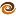EmptyCrate

About Me | Contact Me | Training |ChaiScript.com | YouTube Channel

The follow code demonstrates a method for generating the Fibonacci Sequence at compile time. Since the sequence is generated at compile time runtime execution of retrieving a number is constant time.

There is a recursive generation of templated types in this code such that the instantiation of a template: Fib<10> f; generates 11 classes, namely: Fib<0> through Fib<10>.

The nature of the class generation by the compiler means that previous Fibonacci values are effectively cached, making the compile time go much faster. Therefore, this is pretty much the fastest Fibonacci generator I have seen, even if you include compile time.

#include <iostream>
#include <cassert>
using namespace std;

template<int stage>
struct Fib
{
//Make this value a constant value equal to the (stage-1) + (stage -2)
//which the compile will generate for us and save in the types of:
// Fib<stage-1> and Fib<stage-2>. This all works because stage is known at compile
// time, as all template parameters must be.
static const uint64_t value = Fib<stage-1>::value + Fib<stage-2>::value;

static inline uint64_t getValue(int i)
{
if (i == stage) // Does the current class hold the given place?
{
return value;  // Return it!
} else {
return Fib<stage-1>::getValue(i); // Get it from the previous class!
}
}
};

template<> // Template specialization for the 0's case.
struct Fib<0>
{
static const uint64_t value = 1;

static inline uint64_t getValue(int i)
{
assert(i == 0);
return 1;
}
};

template<> // Template specialization for the 1's case
struct Fib<1>
{
static const uint64_t value = 1;

static inline uint64_t getValue(int i)
{
if (i == 1)
{
return value;
} else {
return Fib<0>::getValue(i);
}
}
};

int main(int, char *[])
{
//Generate (at compile time) 100 places of the Fib sequence.
//Then, (at runtime) output the 100 calculated places.
//Note: a 64 bit int overflows at place 92
for (int i = 0; i < 100; ++i)
{
cout << "n:=" << i << " => " << Fib<100>::getValue(i) << endl;
}
}
My Videos

Related